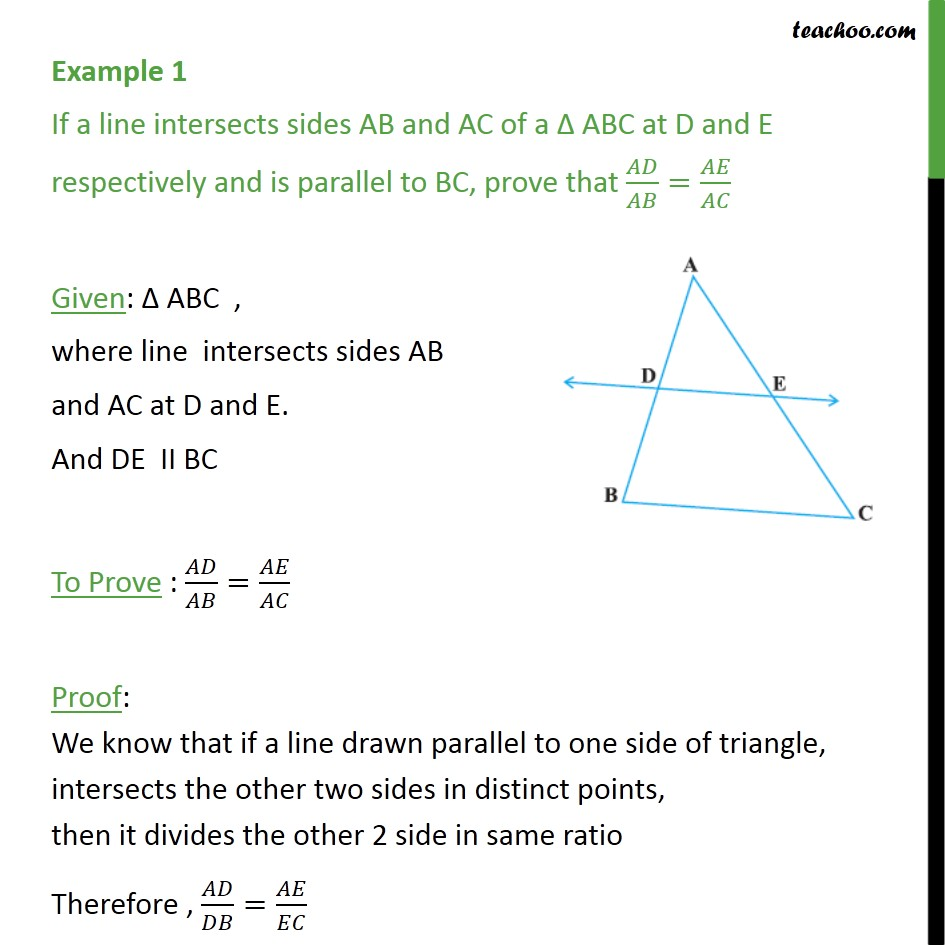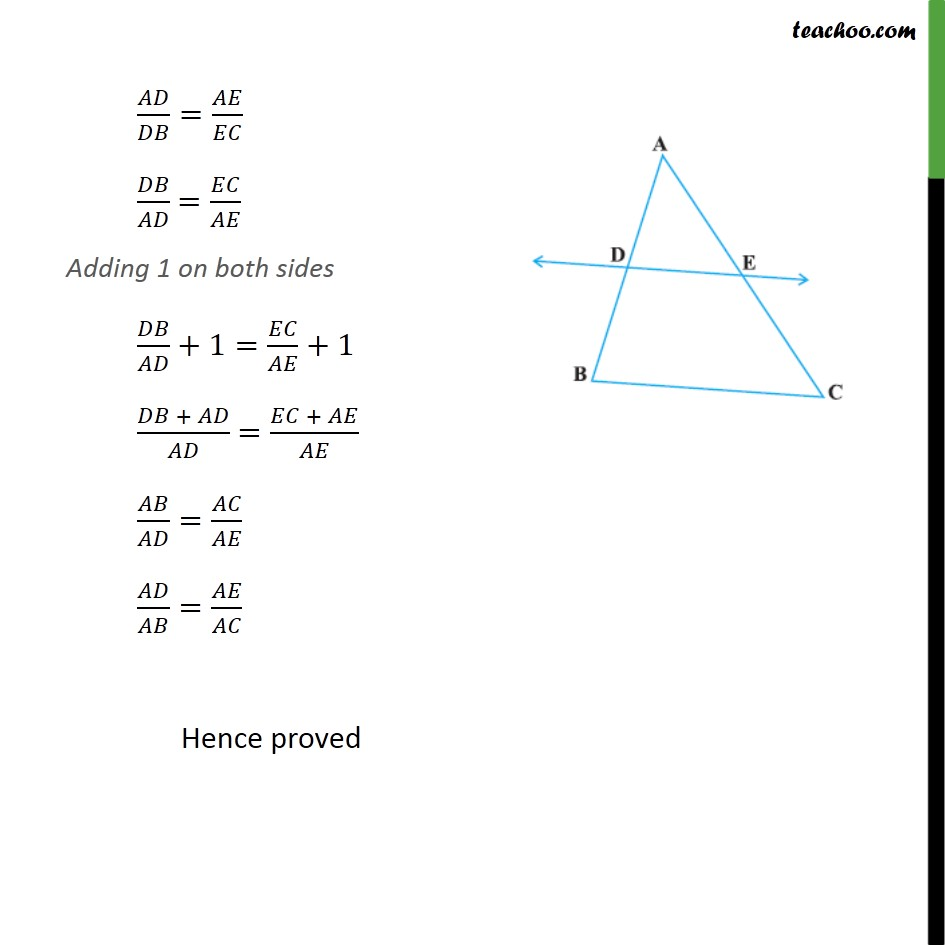Subscribe to our Youtube Channel - https://you.tube/teachoo

1. Chapter 6 Class 10 Triangles
2. Concept wise
3. Theorem 6.1

Transcript

Example 1 If a line intersects sides AB and AC of a Δ ABC at D and E respectively and is parallel to BC, prove that 𝐴𝐷/𝐴𝐵=𝐴𝐸/𝐴𝐶 Given: Δ ABC , where line intersects sides AB and AC at D and E. And DE II BC To Prove : 𝐴𝐷/𝐴𝐵=𝐴𝐸/𝐴𝐶 Proof: We know that if a line drawn parallel to one side of triangle, intersects the other two sides in distinct points, then it divides the other 2 side in same ratio Therefore , 𝐴𝐷/𝐷𝐵=𝐴𝐸/𝐸𝐶 𝐴𝐷/𝐷𝐵=𝐴𝐸/𝐸𝐶 𝐷𝐵/𝐴𝐷 =𝐸𝐶/𝐴𝐸 Adding 1 on both sides 𝐷𝐵/𝐴𝐷+1=𝐸𝐶/𝐴𝐸+1 (𝐷𝐵 + 𝐴𝐷)/𝐴𝐷 =(𝐸𝐶 + 𝐴𝐸)/𝐴𝐸 𝐴𝐵/𝐴𝐷=𝐴𝐶/𝐴𝐸 𝐴𝐷/𝐴𝐵=𝐴𝐸/𝐴𝐶 Hence proved

Theorem 6.1